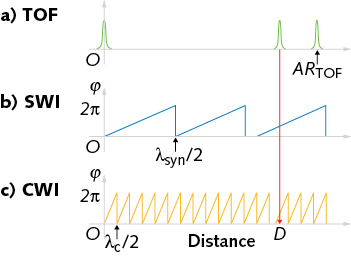# Frequency Combs: Dual-comb instrument precisely finds absolute distance over large range

A distance-measurement system that uses a phase-stable dual-optical frequency comb setup combines time-of-flight measurement, synthetic-wavelength interferometry, and carrier-wave interferometry.The advent of optical frequency combs (OFCs) has led to a number of precision approaches to absolute-distance measurement via interferometry, some using single OFCs and others using a pair of OFCs. However, each of these approaches has some disadvantage, with long averaging times standing out in particular. Shorter averaging times could lead to use of OFC-based interferometers in industry, as well as in more exotic applications such as precise space-satellite formations.

Researchers at Tsinghua University (Beijing and Shenzhen, China) have unveiled just such a distance-measurement system, using a phase-stable dual-OFC setup that combines time-of-flight (TOF) measurement, synthetic-wavelength interferometry (SWI), and carrier-wave interferometry (CWI), all linked together so that they cover for each other’s ambiguities in resolution over their respective ranges, resulting in an overall ambiguity range of 2.67 m (the range within which an unambiguous determination of distance can be found).1

The experimental setup demonstrated a 1.2 µm resolution without time-averaging and a 3 nm resolution with time-averaging over only a 10 ms time span. With time-averaging over 0.1 s or longer, the resolution was less than 1 nm.

### Combining three approaches

The experimental setup contains two OFCs with pulse-train frequencies of 56.090 and 56.092 MHz, respectively, that are mutually locked. The pulse train from comb 1 passes through a Michelson interferometer, then interferes with comb 2. The separate comb 1 and comb 2 are then sampled by a time-shifted version of the comb 2 pulse train, generating reference and measurement interference signals. The distance measurement is calculated as a TOF value that is a function of the time shift, the pulse velocity, the beat frequency, and the frequency of comb 1.

Comb 1 is stabilized in frequency using a piezoelectric transducer and active temperature control, while comb 2 is stabilized by locking the relative frequencies generated by the two combs relative to a free-running continuous-wave (CW) laser emitting at about 1565 nm. The two beat frequencies are electronically mixed to produce a beat signal that is unaffected by noise in the free-running laser.

A high-speed electro-optic phase modulator within the cavity of comb 2 is used to rapidly control the effective cavity length of comb 2. The beat frequency between combs 1 and 2 falls in the radio-frequency (RF) domain; because of the frequency-locking scheme, this RF beat frequency has a sub-hertz linewidth that enables high-precision measurements.

A signal is also constructed to produce a synthetic wavelength of about 76.2 µm. The resulting ambiguity ranges for the three different approaches (TOF, SWI, and CWI) are 2.67 m, 38.1 µm, and 0.773 µm, respectively, while the associated precisions (without averaging) are 8 µm, 1.2 µm, and 19 nm, respectively. When applied together (see figure), the three methods produce a distance measurement unambiguous to the longest ambiguity range, with a precision that is the highest of the three methods (CWI, which has the shortest ambiguity range).A dual-frequency-comb-based interferometer to measure absolute distance combines three different methods: time-of-flight (TOF) measurement, synthetic-wavelength interferometry (SWI), and carrier-wave interferometry (CWI). From the TOF method (a), the multiple integer number of half synthetic wavelengths in the SWI method (b) can be found; similarly, from the SWI method, the multiple integer number of half carrier wavelengths in the CWI method (c) can be found.

While various parameters, such as the reference and measurement carrier phases, drift throughout the experiment, the locking method results in a precision of the carrier phases (without averaging) of only about 0.1 rad, a precision of the carrier phase difference of about 0.15 rad, and a phase precision of the synthetic wavelength of about 0.2 rad.

To determine the measurement accuracy of the setup, a moving retroreflective corner mirror was placed 0.5 m away and moved in 100 µm discrete moving steps over a 1.5 mm range. Combining measurements from the three methods resulted in a data plot of incremental distance vs. displacement in the form of a line with a slope of virtually 1 (the ideal) and deviations (residuals) of less than ±50 nm with a standard deviation of about 30 nm, which the researchers say is mainly caused by environmental disturbance.

The several-meter ambiguity range is combined with a 100 Hz measurement rate; the researchers note that improvements in the SWI portion of the scheme can lead to even higher measurement rates.

### REFERENCE

1. Z. Zhu et al., Opt. Express (2018); https://doi.org/10.1364/oe.26.005747.

More in Test & Measurement
Sponsored